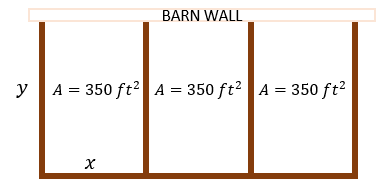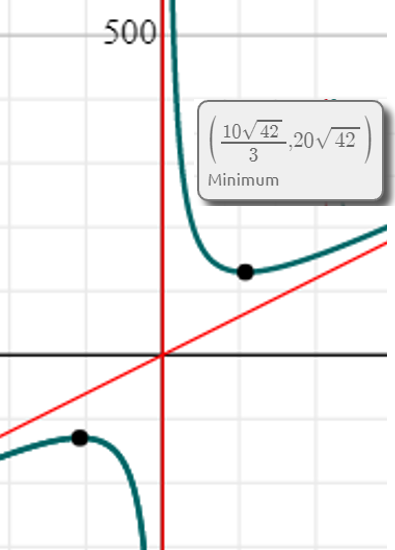# A farmer wishes to fence off three identical adjoining rectangular pens, each with an area of 350...

## Question:

A farmer wishes to fence off three identical adjoining rectangular pens, each with an area of {eq}350 {/eq} square feet, and the entire structure is flushed against the side of his barn. Find the length and width of each pen so that the least amount of fence is required. Prove your answer is a minimum. Sketch and label a diagram at provide appropriate equations.

## Minimum Values:

In dealing with problems that aim to determine the dimensions that would give a minimum or even a maximum value, we need to set-up properly the necessary relations that we can use. After writing down the necessary working equations, we use the specified conditions to know when is the value of the interested variable maximized or minimized.The area of each pen is given by

{eq}\displaystyle A = xy = 350\ \rm ft^2 \Longrightarrow y = \frac{350}{x} {/eq}

Based on the figure above, the total length of the fence needed to enclose the structure is

{eq}\displaystyle P = 3x + 4y = 3x + 4 \bigg( \frac{350}{x} \bigg) = 3x + \frac{1400}{x} {/eq}

We determine the minimum value of {eq}P {/eq}, by plotting its equation.From the plot, we consider the positive dimensions so we take the minimum value of {eq}P_{min} = \bigg(\frac{10 \sqrt{42} }{3} , 20 \sqrt{42} \bigg) {/eq}. This means that the {eq}P {/eq} is minimum when

• {eq}\displaystyle x = \frac{10 \sqrt{42} }{3} \ \rm ft \approx 21.6\ \rm ft {/eq}

At this value of * {eq}x {/eq}, the dimension of * {eq}y {/eq} is

• {eq}\displaystyle y = \frac{350}{x} = \frac{350}{ \frac{10 \sqrt{42} }{3} } \approx 16.2\ \rm ft {/eq}Minimum Values: Definition & Concept

from

Chapter 18 / Lesson 16
145K

The minimum value of a quadratic function is the low point at which the function graph has its vertex. This lesson will define minimum values and give some example problems for finding those values. A quiz will complete the lesson.